Courses

# RD Sharma Solutions -Ex-5.1, (Part - 2) Factorization Of Algebraic Expressions, Class 9, Maths Class 9 Notes | EduRev

## Class 9 : RD Sharma Solutions -Ex-5.1, (Part - 2) Factorization Of Algebraic Expressions, Class 9, Maths Class 9 Notes | EduRev

The document RD Sharma Solutions -Ex-5.1, (Part - 2) Factorization Of Algebraic Expressions, Class 9, Maths Class 9 Notes | EduRev is a part of the Class 9 Course RD Sharma Solutions for Class 9 Mathematics.
All you need of Class 9 at this link: Class 9

Q20 . x2−y2−4xz+4z2

SOLUTION :

On rearranging the terms

= x2−4xz+4z2−y2

= (x)2−2×x×2z+(2z)2−y2

Using the identity x2−2xy+y2=(x−y)2

= (x−2z)2−y2

Using the identity  p2−q2= ( p + q )( p – q )

= (x−2z+y)(x−2z−y)

∴ x2−y2−4xz+4z= (x−2z+y)(x−2z−y)

Q21 .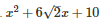SOLUTION :

Splitting the middle term ,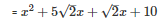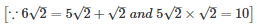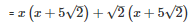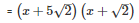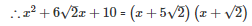Q22 .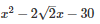SOLUTION :

Splitting the middle term,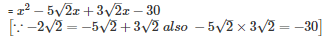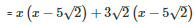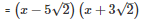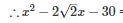=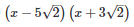Q23 .SOLUTION :

Splitting the middle term,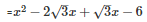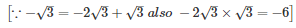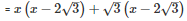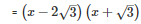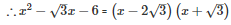Q24 .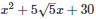SOLUTION :

Splitting the middle term,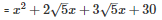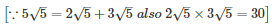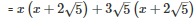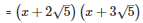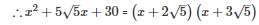Q25 .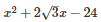SOLUTION :

Splitting the middle term,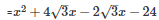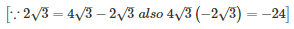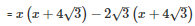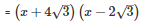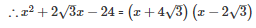Q26 .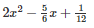SOLUTION :

Splitting the middle term,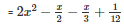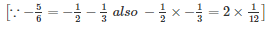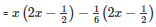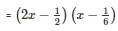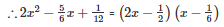Q27 .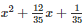SOLUTION :

Splitting the middle term,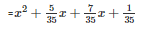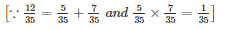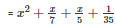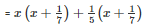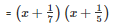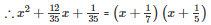Q28 .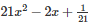SOLUTION :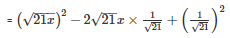Using the identity  (x−y)2=x2+y2−2xy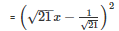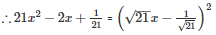Q29 .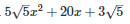SOLUTION :

Splitting the middle term,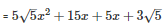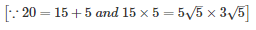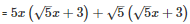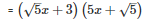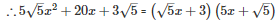Q30 .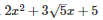SOLUTION :

Splitting the middle term,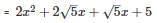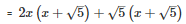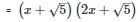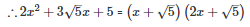Q31 . 9(2a−b)2−4(2a−b)−13

SOLUTION :

Let  2a – b = x

=  9x2−4x−13

Splitting the middle term,

=  9x2−13x+9x−13

=  x(9x−13)+1(9x−13)

=  (9x−13)(x+1)

Substituting  x = 2a – b

=  [9(2a−b)−13](2a−b+1)

=  (18a−9b−13)(2a−b+1)

∴ 9(2a−b)2−4(2a−b)−13 = (18a−9b−13)(2a−b+1)

Q 32 . 7(x−2y)2−25(x−2y)+12

SOLUTION :

Let  x-2y = P

=  7P2−25P+12

Splitting the middle term,

=  7P2−21P−4P+12

=  7P(P−3)−4(P−3)

=  (P−3)(7P−4)

Substituting  P = x – 2y

=  (x−2y−3)(7(x−2y)−4)

=  (x−2y−3)(7x−14y−4)

∴ 7(x−2y)2−25(x−2y)+12 = (x−2y−3)(7x−14y−4)

Q33 . 2(x+y)2−9(x+y)−5

SOLUTION :

Let  x+y = z

=  2z2−9z−5

Splitting the middle term,

=  2z2−10z+z−5

=  2z(z−5)+1(z−5)

=  (z−5)(2z+1)

Substituting  z = x + y

=  (x+y−5)(2(x+y)+1)

=  (x+y−5)(2x+2y+1)

∴ 2(x+y)2−9(x+y)−5 = (x+y−5)(2x+2y+1)

Q34 . Give the possible expression for the  length  &  breadth of the rectangle having   35y2−13y−12 as its area.

SOLUTION :

Area is given as 35y2−13y−12

Splitting the middle term,

Area =  35y2+218y−15y−12

=  7y(5y+4)−3(5y+4)

=  (5y+4)(7y−3)

We also know that area of rectangle  = length ×breadth

∴ Possible length = (5y+4) and  breadth=(7y−3)

Or  possible length = (7y−3) and  breadth= (5y+4)

Q35 . What are  the possible expression for the cuboid having volume 3x2−12x.

SOLUTION :

Volume =  3x2−12x

= 3x(x−4)

= 3×x(x−4)

∴ Possible expression for dimensions of cuboid are = 3 , x , (x−4)

Offer running on EduRev: Apply code STAYHOME200 to get INR 200 off on our premium plan EduRev Infinity!

91 docs

,

,

,

,

,

,

,

,

,

,

,

,

,

,

,

,

,

,

,

,

,

,

,

,

,

,

,

,

,

,

;